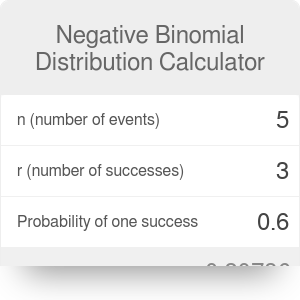n (number of events)
r (number of successes)
Probability of one success
Probability of Y=n

# Negative Binomial Distribution Calculator

By Bogna Haponiuk

This negative binomial distribution calculator, otherwise called the Pascal distribution calculator, can help you determine what is the probability of requiring n trials to achieve a fixed number of successes. We will also provide you with a list of examples of negative binomial distribution to make sure you understand this topic correctly.

## What is the negative binomial distribution?

Both the binomial and negative binomial distribution concern consecutive events with fixed probability of success.

In the binomial distribution, you determine the probability of a certain number of successes observed in n trials. In negative binomial distributions, on the other hand, your random variable is the number of trials needed to get r successes.

Picture it like this: you are handing out leaflets on a street. You have 15 leaflets on you and cannot stop until you give away all of them. The probability that a passerby will take a leaflet from you is 0.4.

• The probability of getting rid of n leaflets if you try to hand them out to 50 people in binomial distribution is P(n).
• The probability of having to try m times to give out a leaflet to get rid of all 15 of them is a negative binomial distribution in P(m).

Notice that while the binomial distribution allows you to try only a specific number of times, the negative has a tail at infinity. After all, you might just have a terrible luck and never give out the last leaflet.

To sum up, in a binomial distribution, you have:

• Fixed number of trials (n);
• Random variable the number of successes (denoted with X);
• Possible values are 0 ≤ X ≤ n.

In the negative binomial distribution, you have:

• Fixed number of successes (r);
• Random variable the number of trials until the r-th success (denoted with Y);
• Possible values are Y ≥ r.

## Negative binomial distribution examples

Some examples of negative binomial distribution include:

• How many times you need to roll a die until you get three results of 6;
• How many times you need to knock on doors during the Halloween night until you collect 20 candy bars;
• How many times you need to flip a coin to get four heads;
• How many attempts you need to score three goals in a match.

## The negative binomial distribution formula

Our negative binomial calculator uses the following formula:

`P(Y=n) = (n-1)C(r-1) * p^r * (1-p)^(n-r)`

where:

• n is the total number of trials;
• r is the number of successes;
• p is the probability of one success;
• (n-1)C(r-1) is the number of combinations (so-called "n choose r"), using the values (n-1) and (r-1);
• P(Y=n) is the probability of the exact number of trials n needed to achieve r successes.

## How to use the Pascal distribution calculator

Let's solve the problem of the leaflets together.

1. Determine the number of successes. r is equal to 15, as we have to hand out 15 leaflets.
2. Determine the number of trials. Let's say you want to calculate the probability of handing all leaflets out in 25 trials.
3. The probability of a stranger taking a leaflet from you is equal to 0.4. Hence, the probability of an individual success p = 0.4.
4. Calculate the number of combinations (n-1 choose r-1, so 24 choose 14). You can use the combination calculator to do it. This number in our case is equal to 1,961,256.
5. Substitute all these values into the binomial probability formula above:

`P(Y=50) = 1,961,256 * 0.4^15 * (1-0.4)^(25-15) = 0.01273`

You can also save yourself some time and use the negative binomial distribution calculator instead :)

Bogna Haponiuk

## Get the widget!

Negative Binomial Distribution Calculator can be embedded on your website to enrich the content you wrote and make it easier for your visitors to understand your message.

It is free, awesome and will keep people coming back!